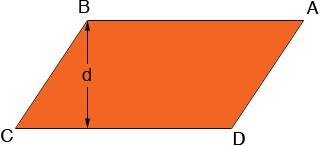SEARCH HOMEMath Central Quandaries & QueriesQuestion from jhon, a student: Calculate the perpendicular distance between AB and DC of a parallelogram. Note: AB and DC are the sides of the parallelogram.Jhon,

What do you know about the parallelogram? You need to know more than the lengths of the sides.For example if you know the length of the side BC and the measure of the angle at C, and d is the perpendicular distance between AB and CD then sin C = d/|BC| so d = |BC| sin C.

I hope this helps,
PennyMath Central is supported by the University of Regina and The Pacific Institute for the Mathematical Sciences.